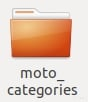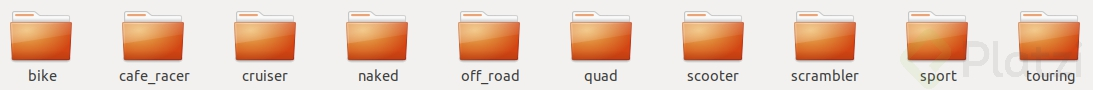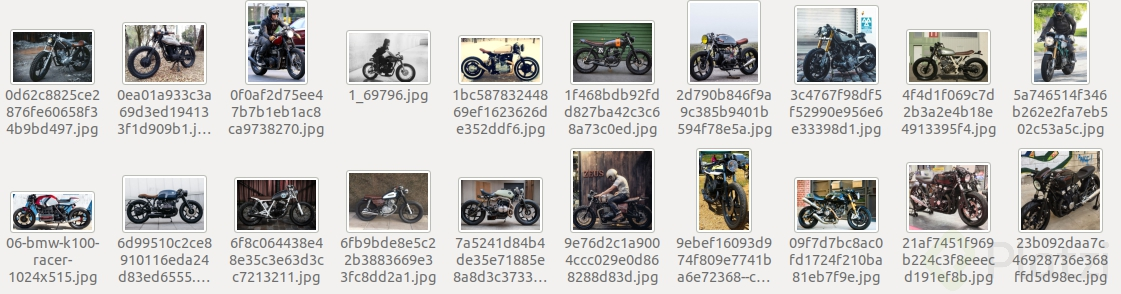8

# Everything is ok but.... How do I create my own dataset?

ricardomvp
22120

If you have a basic or medium Supervised_Learning level and you have worked with pre-load datasets as IRIS, MNIST, SIGNS, Boston Housing, Wine quality, Titanic etc.
And now you are like “everything is OK but… How do I create my own dataset?”. That’s why we are here!

## Define our topic

May be there is a similar topic dataset in a platform like kaggle, but in this moment we don’t care! We want to create our own!.

In our example we decided to work with motorcycles categories

Start creating the root folder:## Define our categories

In this case 8 category:

• Sport
• Cruiser
• Touring
• Naked
• Scrambler
• Cafe racer
• Scooter

And in additional two categories more no properly defined as motorcycles:

• Bike

That means a folder by cat:## Dirty work

Now it is time to “dirty work”, in this case we have to search for images in every category, we can start with minimum 100 images per category:That means make a minimum collection of 1,000 images !!! 😱😱😱

## Start to code

I think it is obvious but we work with Python3

<h3>Create a dict</h3>
``````import os
import random
#Make dict with all images path and image category# stablis folder path
base_folder = 'SOME_PATH/moto_categories'#Make a list with the name of sub-folder name
motos_cathegory = []
for moto in os.listdir(base_folder):
motos_cathegory.append(moto)

#Create dict
all_imgs = []
#For each categoryfor moto in motos_cathegory:
type_moto_folder = base_folder + '/' + moto
#For each image in each categoryfor i, img in enumerate(os.listdir(type_moto_folder)):
path = type_moto_folder + '/' + img
img_dict = {}
img_dict['path'] = path
for m in motos_cathegory:
img_dict[m] = 0
if m == moto:
img_dict[m] = 1
all_imgs.append(img_dict)

#Labelsprint(motos_cathegory)
# all_imgs lenghtprint(len(all_imgs))
``````

This piece of code returns a list of dicts with the following structure:

{‘path’:‘IMAGE_PATH’,
‘naked’: *,
‘scooter’: *,
‘scrambler’: *,
‘cafe_racer’: *,
‘cruiser’: *,
‘sport’: *,
‘touring’: *,
‘bike’: *,
}
. * int(0 or 1) depends on cat

<h3>Randomizing list</h3>

Due to the list was adding element by category the list is in order, we need this list mixed

``````#Randomizing all_imgs listrandom.shuffle(all_imgs)
``````
<h3>Preview</h3>

This is not mandatory but if you want you can establish an image previewer

``````#Librariesimport matplotlib.pyplot as plt
import PIL
from PIL import Image
#Show a random imagen inside all_imgs#Set image size
SIZE=64# random num in the list
num=random.randint(0,len(all_imgs)-1)
``````
``````#Show image
img = Image.open(all_imgs[num]['path'])
img.thumbnails((SIZE, SIZE))
plt.figure()
plt.imshow(img)
#Show cathegory
print(all_imgs[num]['cath'])
``````
<h3>Creating train and test dataset</h3> <h4>Image Peprocessing</h4>

This is not a topic we’re going to dive into in deep but the “processing” are transformations the images suffer in order to create a more balanced image also reduce quality and size and at least transform to tensor.

``````#Torchimport torch
import torchvision
from torchvision import transforms
#Image transformationspreprocess = transforms.Compose ([
transforms.Resize(64),
transforms.CenterCrop(64),
transforms.ToTensor(),
transforms.Normalize(
mean = [0.485, 0.456, 0.406],
std = [0.229, 0.224, 0.225]
)
])
``````
<h4>Data augmentation</h4>

Data augmentation is also image processing but it is used like a technique to grow up our training dataset making some movements in the images in order o look like different for the model.
in our case we will make a a movement in the images well known as mirror.

``````data_augmentation_preprocess = transforms.Compose ([
transforms.RandomHorizontalFlip(p=1),
])
``````
<h4>Define train and test size</h4>
``````train_size = 0.9train_num = int(len(dataset)*train_size)
train = dataset[:train_num]
test = dataset[train_num:]
print(f'len_train = {len(train)}, len_test = {len(test)}')
``````
<h4>Append tensor- label into datasets</h4>

In this part, to be more explicit how to create train and test dataset we follow this flow process:
Train_dataset
Call image -> Prepossessing img -> From result of preprocessing Flat tensor img -> establish category label -> append into a list a tuple of (Tensor,int)
Data augmentation
Prepossessing img (data augmentation)-> From result of preprocessing Flat tensor img -> establish category label -> append into a list a tuple of (Tensor,int)

Test_dataset
same process without Data augmentation

``````##create train dataset##
train_dataset=[]
forimagein train:
#'''Call image'''
img = Image.open(image['path']).convert('RGB')
#'''transform image'''
img_t = preprocess(img)
#'''Flat image tensor'''
batch = torch.unsqueeze(img_t, 0)

#'''Transform moto_cathegory to a int between 0-9
temp=None
for i,cathegory in enumerate(motos_cathegory):
ifimage[cathegory] == 1:
temp=i
break
#Append tuple of image in tensor form and moto cathegory
train_dataset.append((batch,temp))

#Data Augmentationif data_augmentation==True:
img_t2 = data_augmentation_preprocess(batch)
batch2 = torch.unsqueeze(img_t2, 0)
train_dataset.append((batch2, temp))
#Mixing datasetrandom.shuffle(train_dataset)

##create test dataset##
test_dataset=[]
forimagein test:
img = Image.open(image['path']).convert('RGB')
img_t = preprocess(img)
batch = torch.unsqueeze(img_t, 0)

temp=None
for i,cathegory in enumerate(motos_cathegory):
ifimage[cathegory] == 1:
temp=i
break

test_dataset.append((batch,temp))
``````

This piece of code returns two lists train_dataset and test_dataset each list contains for every image a tuple like this (image_tensor, int).

<h3>Congrats 🥳</h3>